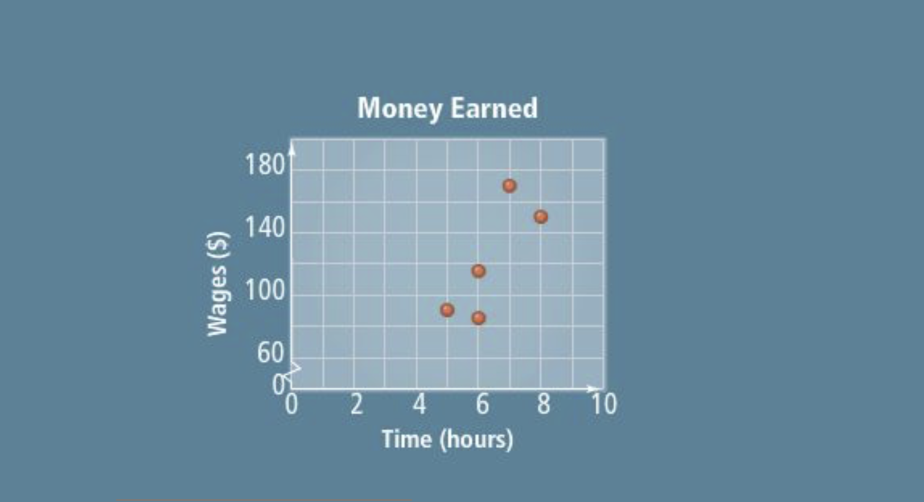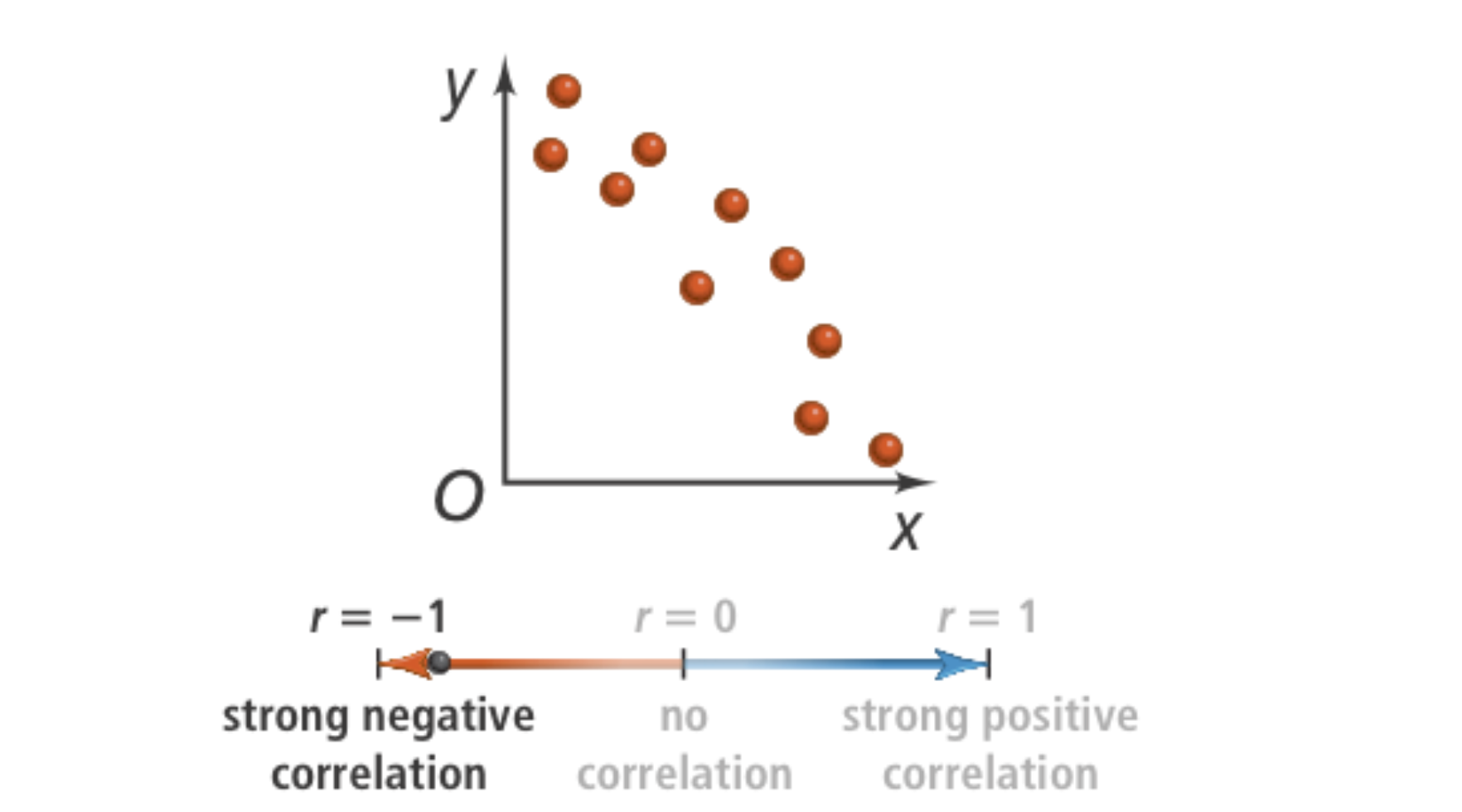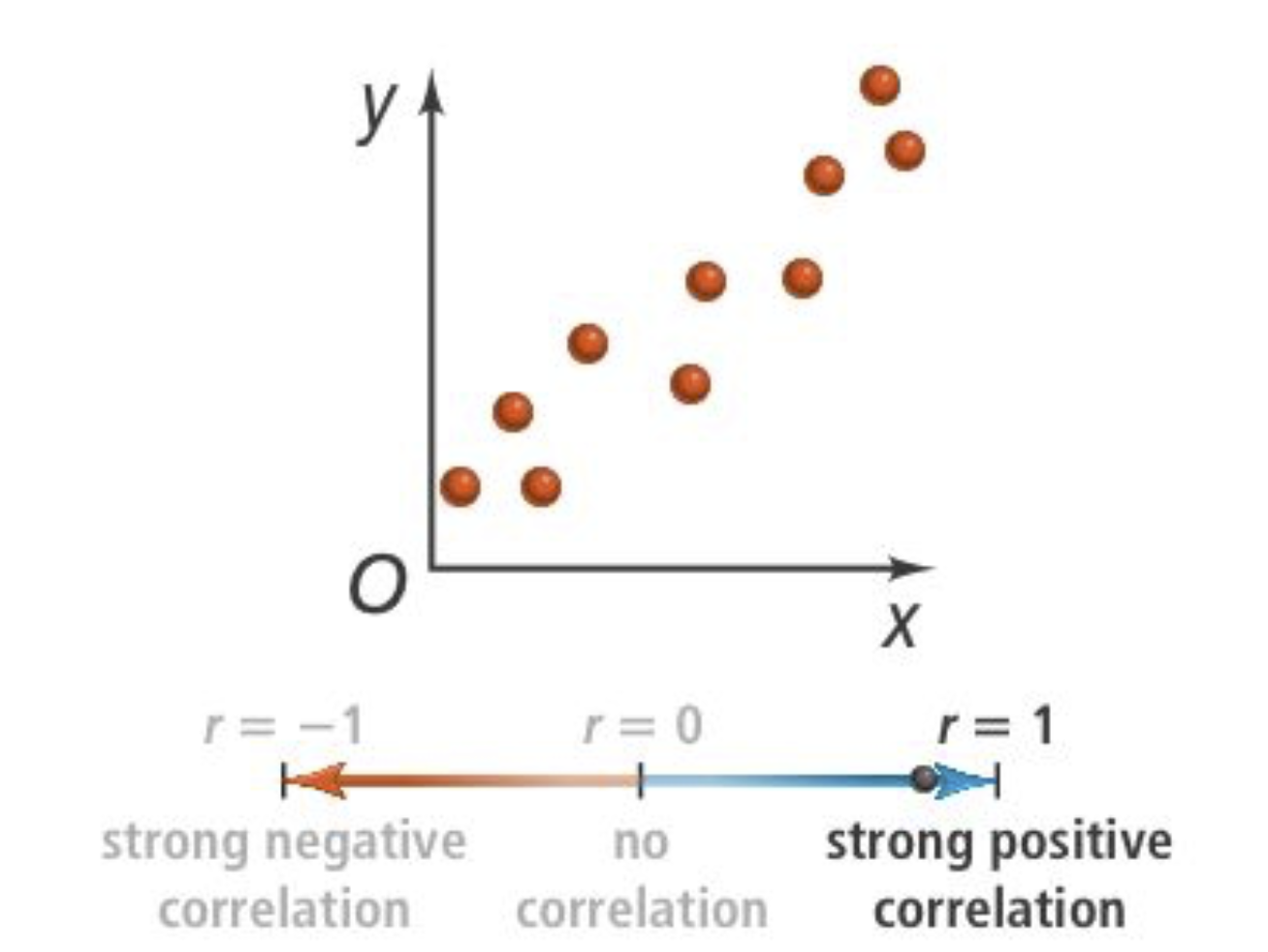Algebra 2 2-5 Guided Practice: Using Linear Models
starstarstarstarstarstarstarstarstarstar
by Matthew Richardson
| 8 Questionsa
1
2
Solve It! The graph shows the number of hours and the amount of money you earned each day last week.
1
10
How many hours should you work to earn \$200? Enter only a number. Your response is only an approximation based on the trend of the data in the graph.
2
103
20
Problem 1 Got It? The table shows the numbers of hours students spend online the day before a test and the scores on the test. Make a scatterplot of these data on the canvas. Use colors that stand out and include all relevant graph detail.

4
10
Problem 1 Got It? How would you describe the correlation between the variables in the table?

Problem 1 Got It? Now use the embedded Desmos graphing utility below to plot the same data.

1. Click the + button and add a table.
2. Input the hours from the table into the x₁ column.
3. Input the test scores from the table into the y₁ column.
4. Zoom and pan your graph to establish a suitable viewing window. (You may use the wrench button for fine tuning.)
5. Take a screenshot of your scatterplot.

5
10
Problem 1 Got It? Upload the screenshot you took of your Desmos scatterplot to the canvas.
6
10
Problem 1 Got It? Reasoning: Using the graph from Problem 1, how much would you expect to pay for electricity if the average temperature was 70°F? Explain.7
7
10
Problem 2 Got It?
A
B
C
D8
8
10
Problem 3 Got It? Consider using the embedded Desmos graphing utility above.
A
B
C
D